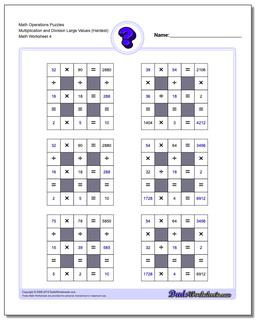PLEASE GO BACK AND USE THE BIG BLUE 'PRINT' BUTTON ON THE PAGE TO PRINT THE WORKSHEET CORRECTLY!Sorry for the trouble! The browser won't print the embedded worksheet PDF directly using the normal 'Print' command in the file menu, so you need to click the big 'Print' button to send just the worksheet and not the surrounding page to the printer.

Math Worksheets: Number Grid Puzzles: Number Grid Puzzles: Math Operations Puzzles Multiplication and Division Large Values (Hardest) (Fourth Worksheet)Math Operations Puzzles Multiplication and Division Large Values (Hardest) (Fourth Worksheet)

PropertyValue
DescriptionMath Operations Puzzles Multiplication and Division Large Values (Hardest): Math puzzle worksheets that require students to fill in missing numbers with values. These worksheets have larger values for the operands and the products than the ones above. (Fourth Worksheet)
Resource TypeWorksheet